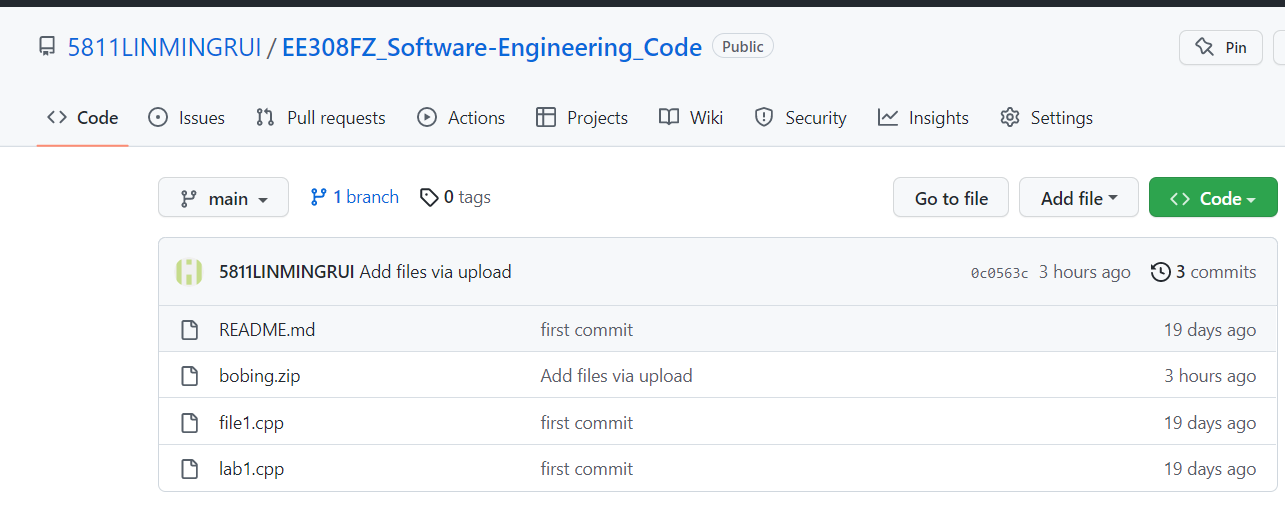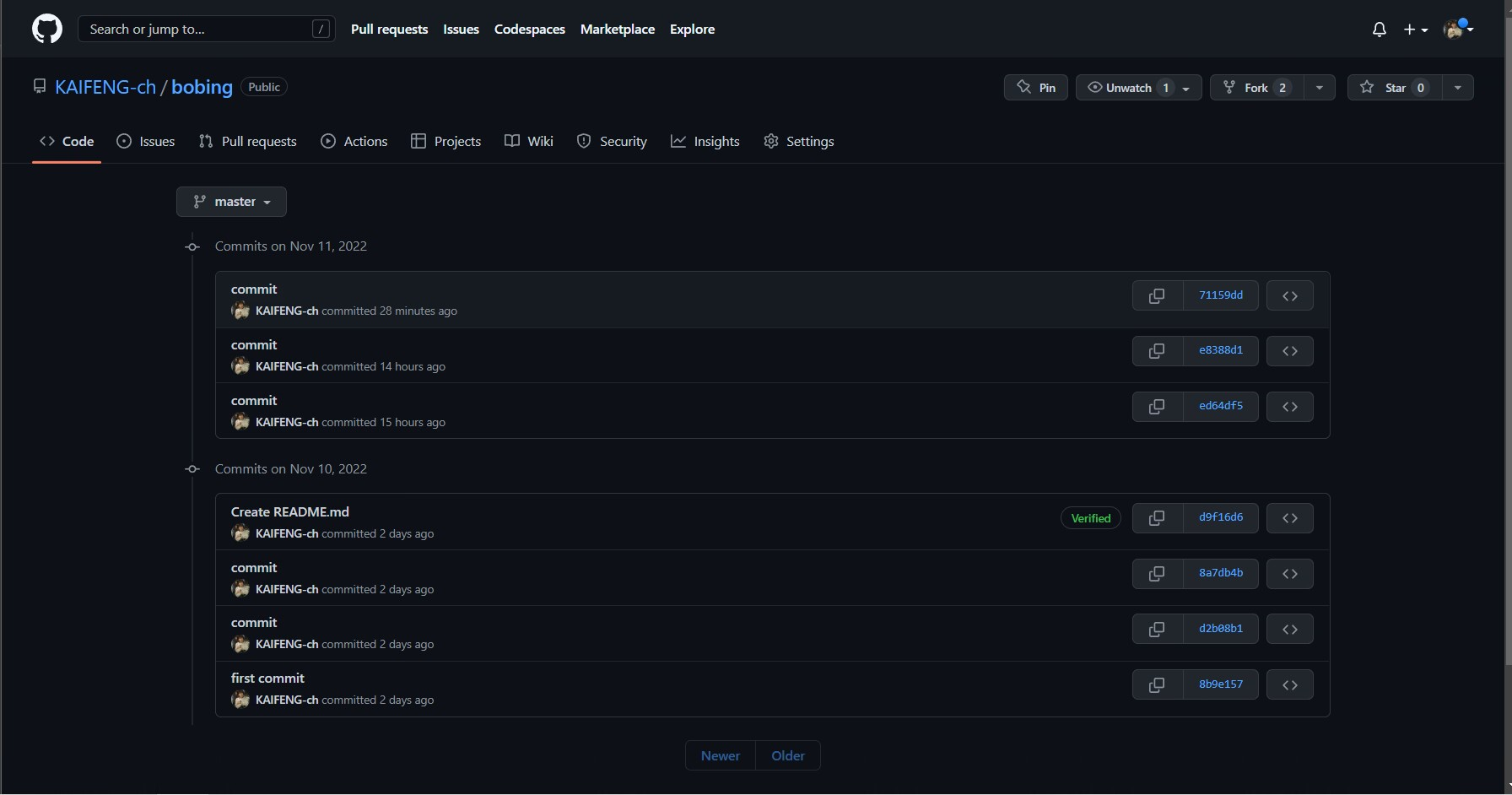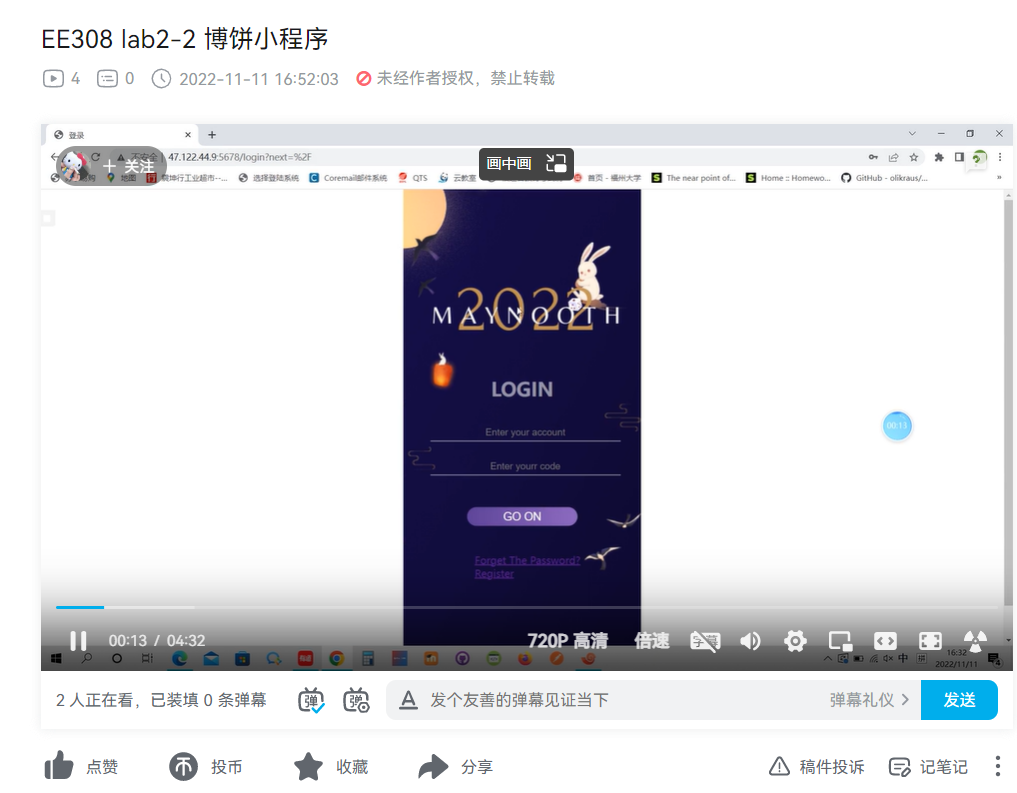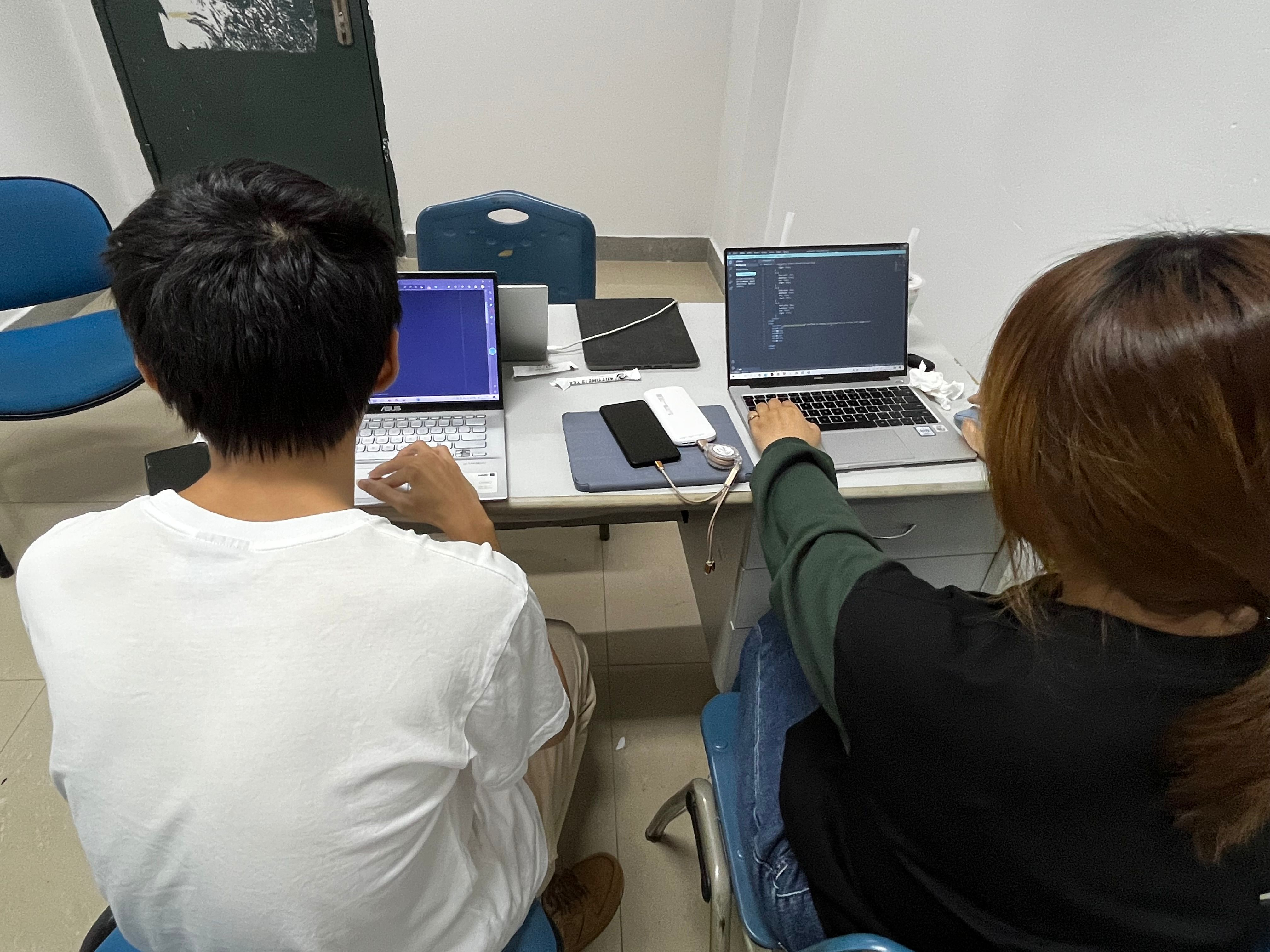# EE308FZ_Lab2-2_832001302_20123108

 The Link Your Class https://bbs.csdn.net/forums/MUEE308FZU202201 The Link of Requirement of This Assignment https://bbs.csdn.net/topics/608859318 MU STU ID and FZU STU ID FZU ID : 832001302    MU ID : 20123108 Teammate's MU STU ID and FZU STU ID MU ID ： 20124732  20122292      FZU ID ：832001124  832001321 Teammate's blog Link GitHub Link https://github.com/5811LINMINGRUI/EE308FZ_Software-Engineering_Code Video demo Link https://www.bilibili.com/video/BV1gd4y1b7Hq/?spm_id_from=333.999.0.0&vd_source=221825654697daa6d6cfd24219ca8ed1 Online Game Link http://47.122.44.9:5678/

### Content

Part 1  :  PSP Table

Part 2  :  Key Function and Program Thinking

Part 3  :  Thing Take Long Time to Finish

Part 4  :  GitHub Link and Commit Record

Part 5  :  Video Demonstration of Software Running

Part 6  :  Paired Work Photo

Part 7  :  Program Experience and Great Gain

### PSP Table

 PSP ESTIMATE TIME（MINUTE） ESTIMATE TIME（MINUTE） Planning 45 30 Estimate 5 5 Development 30 50 Analysis 60 70 Design Spec 10 10 Design Review 0 0 Coding Standard 20 15 Design 40 60 Coding 240 300 Code Review 10 15 Test 60 80 Reporting \ \ Test Report 10 20 Size Measurement 10 10 Postmortem & Process Improvement Plan 20 50 Total 560 715

### Key Function and Program Thinking

•  the back-end programs used to judge logins and calculate scores

This part is about how to use python flask to reach the register and login function, we need to insert the user message to the database, and need to encrypt the password. And when user login, the hashed password need to be compared with the input password. Then we make session to record the user message.

from werkzeug.security import generate_password_hash, check_password_hash
flash
from wtforms import StringField, PasswordField, SubmitField
from wtforms.validators import DataRequired, Length, EqualTo, Regexp
from datetime import timedelta
import logging
import conf
from basic_user import get_user, create_user
from user_info import User
cursor = conf.db.cursor()
app.secret_key = 'kaifeng'
"""登录表单类"""
email = StringField("Email", validators=[DataRequired(), Length(1, 64)])
validators=[
DataRequired(),
Length(1, 64),
Regexp(
"^[A-Za-z][A-Za-z0-9_.]*$", 0, "Usernames must have only letters, " "numbers, dots or underscores", ), ], ) password = PasswordField( "Password", validators=[ DataRequired(), EqualTo("password_confirmation", message="Passwords must match."), ], ) password_confirmation = PasswordField("Confirm password", validators= [DataRequired()]) submit = SubmitField(label="Register") @login_manager.user_loader # 定义获取登录用户的方法 def load_user(user_id): return User.get(user_id) @app.route('/login', methods=['GET', 'POST']) # 登录 def login(): form = LoginForm() if request.method == 'GET': return render_template('login.html', form=form) emsg = None user_name = form.username.data password = form.password.data user_info = get_user(user_name) # 从用户数据中查找用户记录 if user_info is None: emsg = "please enter username or password" return render_template('login.html', err=emsg) else: user = User(user_info) # 创建用户实体 if check_password_hash(user.password_hash, password): # 校验密码 login_user(user) return redirect(url_for('index')) else: emsg = "username or password error!" return render_template('login.html', err=emsg) @app.route("/register", methods=["GET", "POST"]) def register(): if request.method == 'GET': return render_template('register.html') form = RegisterForm() if not form.username.data or not form.password.data or not form.email.data: emsg = "please enter the message" return render_template('register.html', err=emsg) sql = "insert into users(username, password, email, money) " \ "VALUES ('%s', '%s', '%s', '%d')" % \ (form.username.data, generate_password_hash(form.password.data), form.email.data, 0) try: cursor.execute(sql) conf.db.commit() except AttributeError: conf.e() logging.error(AttributeError) return redirect(url_for('login')) @app.route('/') @login_required def index(): return render_template('index.html') @app.route('/rules', methods=['GET']) @login_required def rules(): return render_template('rules.html') @app.route('/multiplate', methods=['GET']) @login_required def mutiplater(): return render_template('multiplater.html') @app.route('/individual', methods=['GET']) @login_required def individual(): get_point = request.args.get('point') sql = f"select money from users where username = '{current_user.username}'" try: cursor.execute(sql) conf.db.commit() result = cursor.fetchall() if result: plus = result + int(get_point) sql_update = f"update users set money = '{plus}' where username = '{current_user.username}'" cursor.execute(sql_update) conf.db.commit() except Exception as e: conf.e() logging.error(e) return render_template('individual.html') @app.route('/multi', methods=['GET']) @login_required def multi(): 2.2 The method of judging one - person bobing In this part we designed an algorithm based on the rules to calculate the player's score and used ajax to dynamically send the data from the front end to the back end. return render_template('Muliti_Mode.html') @app.route('/triple', methods=['GET']) @login_required def triple(): return render_template('triple.html') @app.route('/ranking', methods=['GET']) @login_required def ranking(): sql = "select username, money from users order by money desc limit 5" try: cursor.execute(sql) conf.db.commit() result = cursor.fetchall() except Exception as e: conf.e() logging.error(e) return render_template('test.html') return render_template('ranking.html', u1=result, m1=result, u2=result, m2=result, u3=result, m3=result, u4=result, m4=result, u5=result, m5=result ) if __name__ == '__main__': app.run(host='0.0.0.0', port=5678, debug=True) • The method of judging one - person bobing In this part we designed an algorithm based on the rules to calculate the player's score and used ajax to dynamically send the data from the front end to the back end. function rollTheDice() { setTimeout(function () { if (document.getElementById("b1").innerText === "Open") { document.getElementById("b1").innerText = "Stop" for (var m=1; m<=6; m++) { document.querySelector(".img"+m).setAttribute("src", "../static/png/tzz.gif") } } else { document.getElementById("b1").innerText = "Open" var randomNumber1 = Math.floor(Math.random() * 6) + 1; var randomNumber2 = Math.floor(Math.random() * 6) + 1; var randomNumber3 = Math.floor(Math.random() * 6) + 1; var randomNumber4 = Math.floor(Math.random() * 6) + 1; var randomNumber5 = Math.floor(Math.random() * 6) + 1; var randomNumber6 = Math.floor(Math.random() * 6) + 1; document.querySelector(".img1").setAttribute("src", "../static/png/dice" + randomNumber1 + ".png"); document.querySelector(".img2").setAttribute("src", "../static/png/dice" + randomNumber2 + ".png"); document.querySelector(".img3").setAttribute("src", "../static/png/dice" + randomNumber3 + ".png"); document.querySelector(".img4").setAttribute("src", "../static/png/dice" + randomNumber4 + ".png"); document.querySelector(".img5").setAttribute("src", "../static/png/dice" + randomNumber5 + ".png"); document.querySelector(".img6").setAttribute("src", "../static/png/dice" + randomNumber6 + ".png"); var result = [randomNumber1, randomNumber2, randomNumber3, randomNumber4, randomNumber5, randomNumber6] var count = [0, 0, 0, 0, 0, 0] var point = 0 for (var i in result) { count[result[i] - 1]++ } console.log(result) console.log(count) if (count === 4 && count === 2) { document.querySelector("h1").innerHTML = "Chajinhua!" point = 12 } if (count === 6) { document.querySelector("h1").innerHTML = "Zhuanyuan(Liubeihong)!" point = 11 } else if (count === 6) { document.querySelector("h1").innerHTML = "Zhuanyuan(Biandijin)!" point = 10 } else if (count === 6) { document.querySelector("h1").innerHTML = "Zhuanyuan(Heiliubo)!" point = 9 } else if (count === 5 && count === 1) { document.querySelector("h1").innerHTML = "Zhuanyuan(Wuhong)!" point = 8 } else if (count === 5) { document.querySelector("h1").innerHTML = "Zhuanyuan(Wuzidengke)!" point = 7 } else if (count === 4) { document.querySelector("h1").innerHTML = "Zhuanyuan(Sidianhong)!" point = 6 } else if (count === 1 && count === 1 && count === 1 && count === 1 && count === 1 && count === 1) { document.querySelector("h1").innerHTML = "Duitang(Bangyang)!" 2.3 Judging function for double bobing In two-player mode, we use a specific algorithm to judge the outcome of the user's game and select a winner. point = 5 } else if (count === 3) { document.querySelector("h1").innerHTML = "Sanhong(Tanhua)!" point = 4 } else if (count === 4) { document.querySelector("h1").innerHTML = "Sijing(Jinshi)!" point = 3 } else if (count === 2) { document.querySelector("h1").innerHTML = "Erju(Juren)!" point = 2 } else if (count === 1) { document.querySelector("h1").innerHTML = "Yixiu(Xiucai)!" point = 1 } else { document.querySelector("h1").innerHTML = "Thanks for participation!" } var data = { point: point } function sendData() {$.ajax({
type: 'GET',
url: '/individual',
data: data,
dataType: 'json',
success: function (data) {},
error: function (xhr, type) {}
})
}
sendData()
}
}, 1000)
}
• Judging function for double bobing In two-player mode

We use a specific algorithm to judge the outcome of the user's game and select a winner.

function open() {
for (var m=1; m<=6; m++) {
document.getElementById("b1").innerText = "Stop"
document.querySelector(".img"+m).setAttribute("src",
"../static/png/tzz.gif")
}
}
function clear() {
document.getElementById("b1").innerText = "Open"
}
function stop(i) {
var randomNumber1 = Math.floor(Math.random() * 6) + 1;
var randomNumber2 = Math.floor(Math.random() * 6) + 1;
var randomNumber3 = Math.floor(Math.random() * 6) + 1;
var randomNumber4 = Math.floor(Math.random() * 6) + 1;
var randomNumber5 = Math.floor(Math.random() * 6) + 1;
var randomNumber6 = Math.floor(Math.random() * 6) + 1;
document.querySelector(".img1").setAttribute("src", "../static/png/dice"
+ randomNumber1 + ".png");
document.querySelector(".img2").setAttribute("src", "../static/png/dice"
+ randomNumber2 + ".png");
document.querySelector(".img3").setAttribute("src", "../static/png/dice"
+ randomNumber3 + ".png");
document.querySelector(".img4").setAttribute("src", "../static/png/dice"
+ randomNumber4 + ".png");
document.querySelector(".img5").setAttribute("src", "../static/png/dice"
+ randomNumber5 + ".png");
document.querySelector(".img6").setAttribute("src", "../static/png/dice"
+ randomNumber6 + ".png");
var result = [randomNumber1, randomNumber2, randomNumber3, randomNumber4,
randomNumber5, randomNumber6]
var count = [0, 0, 0, 0, 0, 0]
for (var j in result) {
count[result[j] - 1]++
}
console.log(result)
console.log(count)
if (count === 4 && count === 2) {
document.querySelector("h" + (i + 1)).innerHTML = "Chajinhua!"
points[i] = 12
}
if (count === 6) {
document.querySelector("h" + (i+1)).innerHTML =
"Zhuanyuan(Liubeihong)!"
points[i] = 11
} else if (count === 6) {
document.querySelector("h" + (i+1)).innerHTML =
"Zhuanyuan(Biandijin)!"
points[i] = 10
} else if (count === 6) {
document.querySelector("h" + (i+1)).innerHTML =
"Zhuanyuan(Heiliubo)!"
points[i] = 9
} else if (count === 5 && count === 1) {
document.querySelector("h" + (i+1)).innerHTML = "Zhuanyuan(Wuhong)!"
points[i]=8
} else if (count === 5) {
document.querySelector("h" + (i+1)).innerHTML =
"Zhuanyuan(Wuzidengke)!"
points[i] = 7
} else if (count === 4) {
document.querySelector("h" + (i+1)).innerHTML =
"Zhuanyuan(Sidianhong)!"
points[i] = 6
}
else if (count === 1 && count === 1 && count === 1 && count
=== 1 && count === 1 && count === 1) {
document.querySelector("h" + (i+1)).innerHTML = "Duitang(Bangyang)!"
points[i] = 5
}
else if (count === 3) {
document.querySelector("h" + (i+1)).innerHTML = "Sanhong(Tanhua)!"
points[i] = 4
}
else if (count === 4) {
document.querySelector("h" + (i+1)).innerHTML = "Sijing(Jinshi)!"
points[i] = 3
}
else if (count === 2) {
document.querySelector("h" + (i+1)).innerHTML = "Erju(Juren)!"
points[i] = 2
}
else if (count === 1) {
document.querySelector("h" + (i+1)).innerHTML = "Yixiu(Xiucai)!"
points[i] = 1
} else {
document.querySelector("h" + (i+1)).innerHTML = "Thanks for
participation!"
points[i] = 0
}
}
// 掷骰子的功能
function rollTheDice() {
setTimeout(function () {
if (document.getElementById("b1").innerText === "Open") {
open()
} else {
for (var i=0; i<2; i++) {
if (points[i] === -1) {
clear()
stop(i)
break
}
}
if (points[points.length-1] !== -1) {
if (points===points) {
document.querySelector("h3").innerHTML = "draw!"
} else {
points > points ?
document.querySelector("h3").innerHTML = "player 1 win!" :
document.querySelector("h3").innerHTML = "player 2 win!"
}
points=[-1,-1]}
}
},1000)
}


### Thing Take Long Time to Finish

1. We spent a lot of time learning about the front end and back end knowledge

As the saying goes: All things are difficult before they are easy. Since we had never come into contact with HTML, CSS, Javascript and other technologies, and also forgot the python we learned in the freshman year, we spent a lot of time at the beginning watching relevant videos on platforms like Bilibili and CSDN, reading related blog materials, mastering some basic knowledge and having a general understanding of the technology. And then learn other advanced knowledge as needed during the implementation of the function.

2. We ran into a lot of difficulties in programming the logic

In the process of software practice, especially the setting of the logic of the game is relatively difficult. Sometimes there are some errors that need to be modified, and sometimes it takes a lot of time to find the errors.

3. Encountered many difficulties while debugging the server

We used a server we had before, but we didn't know why it couldn't access the IP. We searched a lot of information and made a lot of attempts but couldn't access the IP. Then we bought a new server and still didn't solve the problem.

4. Constantly optimizing software takes time

When we finish a version, we find problems that need to be fixed, and then we make suggestions to improve the game interface and the game experience, which sometimes requires rework and takes a lot of time.

### GitHub Link and Commit Record### Video Demonstration of Software Running

https://www.bilibili.com/video/BV1gd4y1b7Hq/?spm_id_from=333.999.0.0&vd_source=221825654697daa6d6cfd24219ca8ed1### Paired Work Photo### Program Experience and Great Gain

Actually, we stayed up a few nights and even consumed some kinds of junk food as supply of body energy hhh~ For us, although it was a really hard process, we learned a lot basic and common grammar usages on developing the applets, which also make us realize the vital importance of communication and cooperation with partners, eventually, we achieve a sense of achievement.

The level of difficulty in this lab is not friendly for us due to the limitation of time and specialized  master of software development. Luckily, since one of our teammates is more familiar with using flask structure, we use it as a tool to develop web service. Meanwhile, for front end, we know more about how to use html and css language, that we never deal with before, to properly arrange a applet web page and use javascript to judge the results of dices; for back end, we use python to check the information of logging and store the account’s data. Furthermore, we successfully package all the program and deliver it to the Aliyun sever. Despite there are a lot of features we haven't implemented yet, we still propose some plans for out further improvement if we had enough time such as improvements of multiplayer interface’s and optimization of the game experience and set up BGM, etc....全文
211 回复 打赏 收藏 举报23-36表格打印文字显示不全是怎么会事.avi
word＋excel＋ppt，使用技巧视频教程，解决你93.5％的办公应用需求我的图书馆管理系统.zipexplain分析sql脑图
explain分析sql具体字段含义脑图23-04饼图中一小块延伸出柱形图怎么做？.avi
word＋excel＋ppt，使用技巧视频教程，解决你93.5％的办公应用需求计算机组成原理之主存储器的基本组成

287